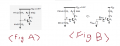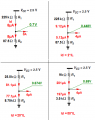# BJT Resistive Divider Biasing

#### HARIBO3

Joined Dec 12, 2018
11
So basically I was studying microelectronics written by Razavi and stucked with the part 5.2.2 (Resistive Divider Biasing)

The text shows 2 examples shown below.It asked the value of Ic and the difference between Fig A and B is that it has a different value of R1 and R2, respectively, which is 17k / 8k and 170k / 80k.

I had no difficulty getting Ic in fig A, since I used a formula Ic = Is*exp(V_be/V_t)

but when it comes to Fig B I went wrong because I_b, the base current, was not negligible and I had to get a V_thev and R_thev.

So I was wondering when should I use the Thevenin equivalent circuit?

and what is the standard to use it, so to say what value of R1 and R2 makes I_b considerable?

#### Jony130

Joined Feb 17, 2009
5,020
So you haven't studied the Thevenin theorem? Or you do not know how to apply it in this specific case?
Simply disconnect the base terminal from the circuit and find the Vth and Rth for a voltage divider alone.

#### HARIBO3

Joined Dec 12, 2018
11
I have studied Thevenin theorem, but I don't know when to apply it.

I'm confused that in Fig B the answer used a thevenin theorem while Fig A didn't.

#### Jony130

Joined Feb 17, 2009
5,020
And you don't know why? Do you know how the base current effect the voltage divider output voltage?

Last edited:

#### HARIBO3

Joined Dec 12, 2018
11
And you don't know why? Do you know how the base current effect the voltage divider output voltage?
From the above circuit output voltage equals to Vcc - IcRc, and Ic equals to beta cross Ib, which concludes that if the base current increases output voltage must be decreased.

(Assuming that output voltage is equal with V_Y)

#### Jony130

Joined Feb 17, 2009
5,020
But how this base current effect the V_X voltage? This is a more important question now. If you want to understand the difference between fig A and fig B.

•LvW

#### HARIBO3

Joined Dec 12, 2018
11
But how this base current effect the V_X voltage? This is a more important question now. If you want to understand the difference between fig A and fig B.
since Ic = Is * exp(V_X / Vt)
Ib = Is * exp(V_X / Vt) / beta

If base current increases so should the V_X increases exponentially

and what's the correlation?

#### Jony130

Joined Feb 17, 2009
5,020
since Ic = Is * exp(V_X / Vt)
Ib = Is * exp(V_X / Vt) / beta

If base current increases so should the V_X increases exponentially

and what's the correlation?

This is only true if you drive you BJT's directly from an ideal voltage source. But in your example, we have a different situation.

Please examine this example very carefully:In which case can we negligible the load current? And why?

#### HARIBO3

Joined Dec 12, 2018
11
Denoting the figure as

A B
C D

every four except b is considered to be negliable.

For case A, the load current does not exist.

For case C and D, the current that flows toward R2 is more than 10 times bigger than the load current.

And we consider the current to be negliable if it's more than 10 times bigger.

For case B we cannot ignore the load current because it has almost the same value with the current that flows toward R2.

#### HARIBO3

Joined Dec 12, 2018
11
thus we can neglect the base current in Fig C and D

#### Jony130

Joined Feb 17, 2009
5,020
•Zeeus

#### HARIBO3

Joined Dec 12, 2018
11
But we don't know whether the load current is negligible BEFORE comparing the load current and the voltage divider current.

Should I get all the values by doing a Thevenin theorem and compare?

#### Jony130

Joined Feb 17, 2009
5,020
But we don't know whether the load current is negligible BEFORE comparing the load current and the voltage divider current.
What you can do is to first ignore the base current and solve directly for Ib. Then we can verify if our assumption was right. And if Ib << Idv = Vcc/(R1 + R2) we are fine and our assumption was right.
If not, we are forced to use Thevenin theorem and recalculate the quiescent current.